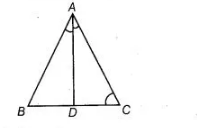# If D is a point on the side BC of a ΔABC`
Question:

If D is a point on the side BC of a ΔABC such that AD bisects ∠BAC. Then,

(a) BD = CD

(b) BA > BD

(c) BD > BA

(d)CD > CA

Thinking Process

(i) Firstly, use the property, exterior angle of a triangle is greater than interior opposite

angle.

(ii) Secondly, use the property that in a triangle, the side opposite to the greater angle is longer.

Solution:

(b) Given, $\triangle A B C$ such that $A D$ bisects $\angle B A C$

$\therefore$ $\angle B A D=\angle C A D$ $\ldots(i)$In $\triangle A C D, \angle B D A$ is an exterior angle.

$\therefore \quad \angle B D A>\angle C A D$ $\ldots(i)$

$[\because$ exterior angle $>$ interior opposite angle]

$\Rightarrow \quad \angle B D A>\angle B A D \quad$ [from Eq. (i)]

$\Rightarrow$ $B A>B D$ [side opposite to greater angle is greater]